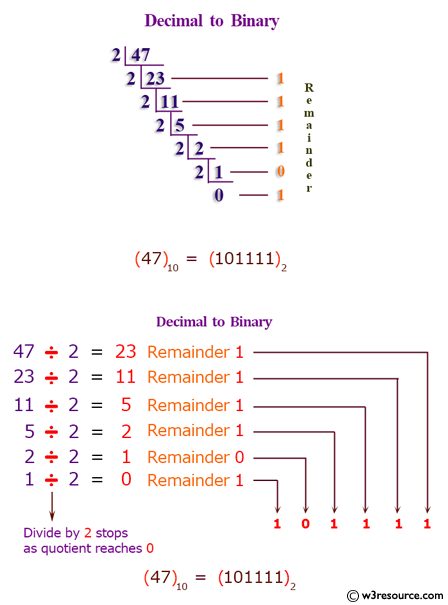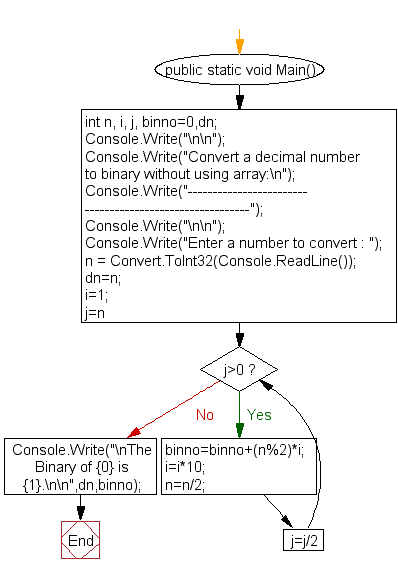﻿ C# - Convert a decimal number to binary without an array# C# Sharp Exercises: Convert a decimal number to binary without using an array

## C# Sharp For Loop: Exercise-41 with Solution

Write a program in C# Sharp to convert a decimal number into binary without using an array.

Pictorial Presentation:Sample Solution:

C# Sharp Code:

``````using System;
public class Exercise41
{
public static void Main()
{
int n, i, j, binno=0,dn;
Console.Write("\n\n");
Console.Write("Convert a decimal number to binary without using array:\n");
Console.Write("---------------------------------------------------------");
Console.Write("\n\n");
Console.Write("Enter a number to convert : ");
dn=n;
i=1;
for(j=n;j>0;j=j/2)
{
binno=binno+(n%2)*i;
i=i*10;
n=n/2;
}

Console.Write("\nThe Binary of {0} is {1}.\n\n",dn,binno);
}
}
```
```

Sample Output:

```Convert a decimal number to binary without using array:
---------------------------------------------------------
Enter a number to convert : 65
The Binary of 65 is 1000001.
```

Flowchart:C# Sharp Code Editor:

Contribute your code and comments through Disqus.

What is the difficulty level of this exercise?

Test your Programming skills with w3resource's quiz.

﻿